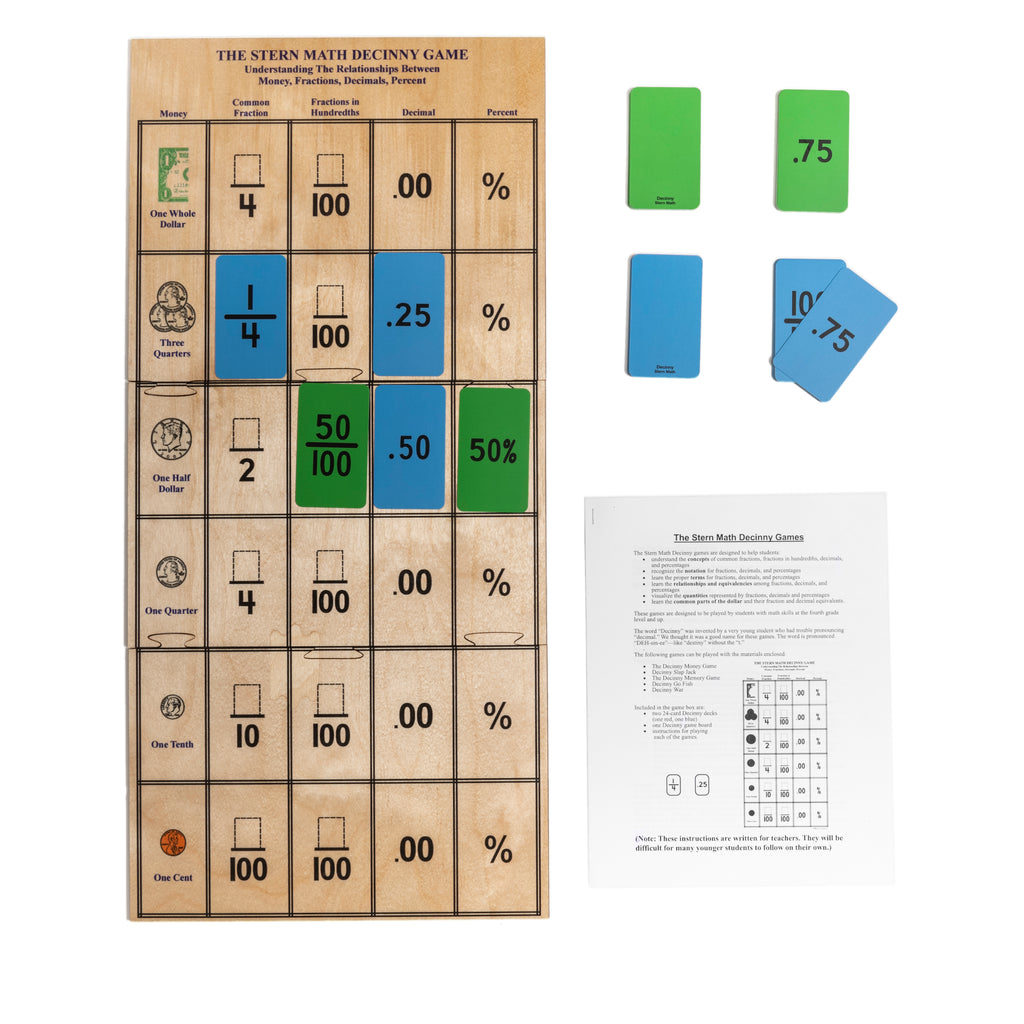# Teaching Numbers: Fractions

## Fraction Blocks

The Complete Fraction Set includes wooden fraction materials which encourage children to discover and become familiar with the concepts of fractions. Children learn equivalence, addition and subtraction, improper fractions, doubling and halving fractions. They also learn to relate fractions to a number line.

This set includes the Core Fractions, Supplemental Fractions, and the book of Fraction Games, which can each also be purchased separately. Fraction Book I is also available for purchase, providing student worksheets that correspond with the fraction sequence and problem solving opportunities for students.The Fraction Blocks are used to teach:

• Fraction concepts and language (numerator, denominator, fraction names)
• Fractions that equal 1 whole
• Comparing, ordering, decomposing fractions
• Equivalent fractions
• Addition and subtraction with fractions
• Fractions of a group
• Improper fractions and mixed numbers
• Problem solving

## Decinny Game

The Stern Math Decinny games are designed to help students understand the concepts and relationships of common fractions, fractions in hundredths, decimals, and percentages as they relate to one another, and to the common parts of a dollar. The multifaceted games help children actively engage with the material and gain mastery and fluency through practice. Once familiar, children can play the games with one another on their own.The Decinny Game is used to teach:

• Relationships between fractions, decimals, money and percents
• Comparing/ordering the different representations of number